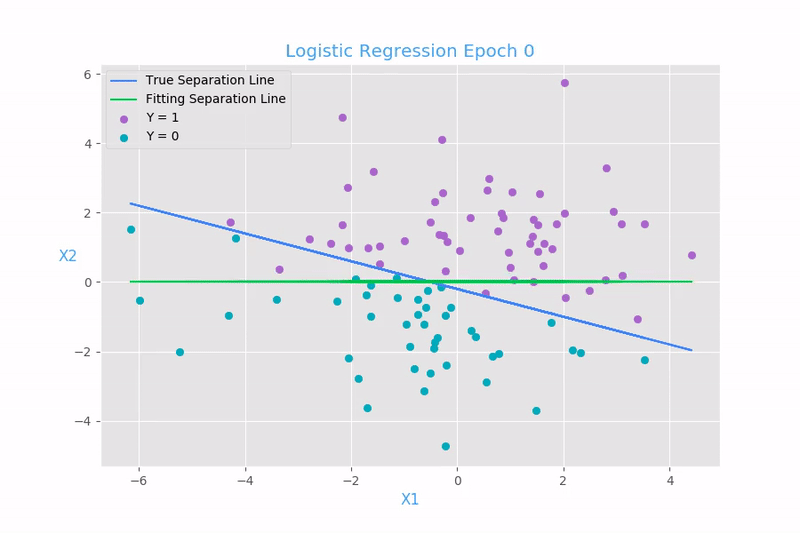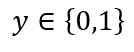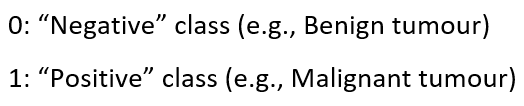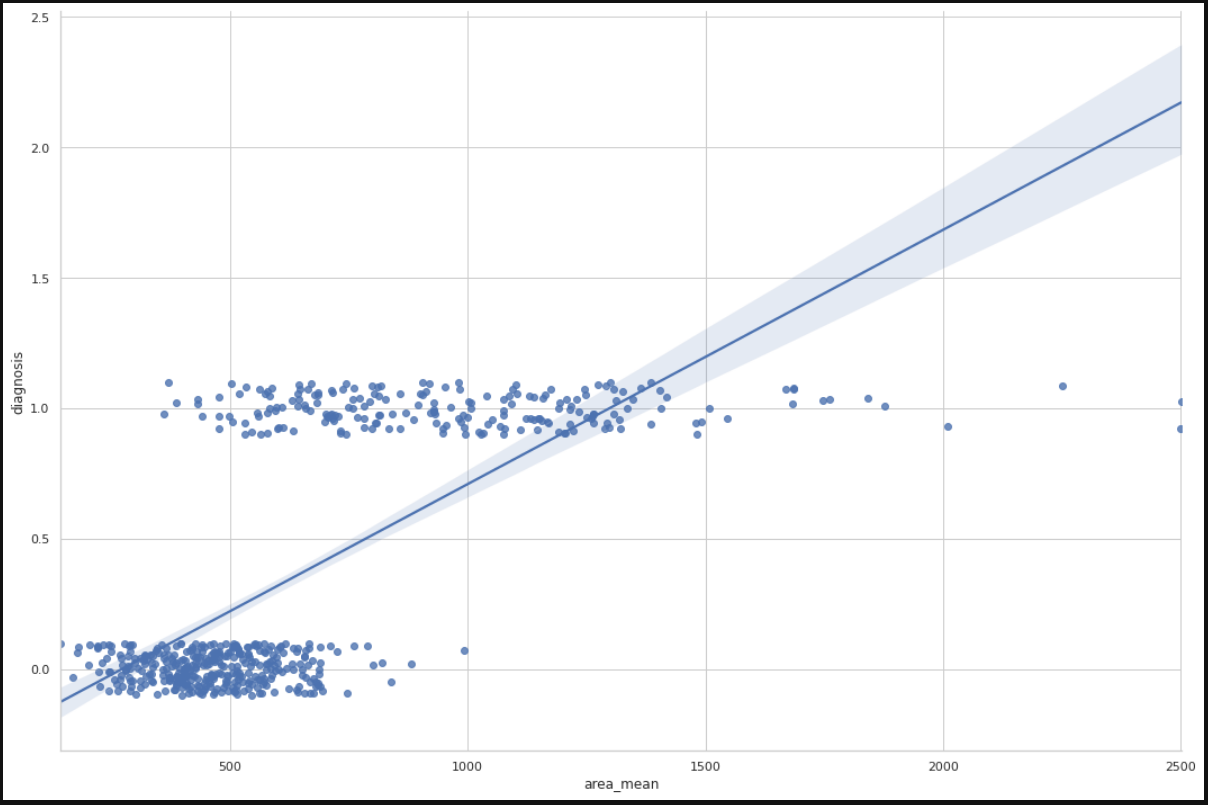1593373440

# Understanding Logistic Regression the Geometric Way

## What is Logistic Regression?

Logistic Regression is one of the basic and popular algorithms to solve a classification problem, unlike its name which has Regression in it. It is named as ‘Logistic Regression’, because its underlying technique is quite the same as Linear Regression. One of the logistic regression’s advantages is because it can handle various types of relationships, not limited to linear relationships.

## Let’s try to understand the Geometric Intuition behind Logistic Regression:

There is more than one approach to understanding logistic regression majorly as Probabilistic ApproachGeometric Approach, and Loss function Minimisation approach, but among all Geometric Approach is what I personally feel is more intuitive to understand. So let’s see :

Assumptions: The underlying assumption for the Logistic Regression is that data is almost or perfectly linearly separable i.e either all (+ve) and (-ve) classes are separated and if not, very few of them are mixed.

Objective: Our objective is to find a plane (**π) **That best separates (+ve) and (-ve) classes.

**Basics: **Let’s have a look at some of the basic terminologies that will make things easier to understand.

We will represent a plane with. Pi(𝜋) and Normal to Plane with** W**

Equation of plane :

Plane Visualisation

w^t*xi+b=0, where b is scalar and xi is the ith observation. and If the plane passes through origin the equation becomes w^t*xi = 0,

Where w^t(read as Wtranspose) is row vector and **xi **is a column vector

## Geometric Interpretation :

Plane separating +ve and -ve classes

If we take any +ve class points, their distance di from the plane is computed as :

(di = w^t*xi/||w||. let, norm vector (||w||) is 1)

Since w and xi in the same side of the decision boundary then distance will be +ve. Now compute dj = w^t*xj since xj is the opposite side of w then distance will be -ve.

we can easily classify the point into -ve and +ve points by using if (w^tx >0) then +ve class and if (w^tx <0) then -ve class

So our classifier is :

``````If w^t * xi > 0  :  then Y = +1    where Y is the class label
If w^t * xi < 0  :  then Y = -1    where Y is the class label
``````

Observations :

Carefully looking at the points on the above diagram we observe the following cases:

``````case 1: Yi >0 and  w^t * xi > 0
Yi = +1 means that the correct class label is +ve => Yi* w^t * xi >0 means that we have correctly predicted the class label.
as +ve * +ve = +ve

case 2: Yi <0 and  w^t * xi <0
Yi = -1 means that the correct class label is -ve => Yi* w^t * xi >0 means that we have correctly predicted the class label.
as -ve * -ve = +ve
case 3: Yi >0 and  w^t * xi <0
Yi = +1 means that the correct class label is -ve => Yi* w^t * xi <0 means that we have wrongly predicted the class label.
as +ve * -ve = -ve
case 2: Yi <0 and  w^t * xi >0
Yi = -1 means that the correct class label is -ve => Yi* w^t * xi <0 means that we have wrongly predicted the class label.
as -ve * +ve = -ve
``````

#algorithms #data-science #logistic-regression #classification-algorithms #machine-learning #algorithms

## Buddha Community1604661480

## Logistic Regression With Geometric Formulation

Logistic Regression is a machine learning technique that is used in classification the name is regression but is actually a classification technique it is used to find the relationship between the dependent and independent variables it is also represented as many names like logit log function etc. it is mostly used in a binary classification where one or more dependent variables are the independent variable

This can be extended, several classes like data set contain images like cat dog and elephant, etc. it assigns the probability between 0 and 1 and adding them, this is a simple explanation about logistic regression so let’s go too deep and try to exact formulation of logistic regression and perform some mathematical operation to option the logistic regression

### Logistic Regression With Geometric Methods

Logistic Regression can be performed by three methods like Geometric probability and loss-function. In this article, we will try to perform a geometrical formulation of logistic regression.

#logistic-regression #geometric #logistic-sigmoid #deep-learning1598352300

## Regression: Linear Regression

Machine learning algorithms are not your regular algorithms that we may be used to because they are often described by a combination of some complex statistics and mathematics. Since it is very important to understand the background of any algorithm you want to implement, this could pose a challenge to people with a non-mathematical background as the maths can sap your motivation by slowing you down.In this article, we would be discussing linear and logistic regression and some regression techniques assuming we all have heard or even learnt about the Linear model in Mathematics class at high school. Hopefully, at the end of the article, the concept would be clearer.

**Regression Analysis **is a statistical process for estimating the relationships between the dependent variables (say Y) and one or more independent variables or predictors (X). It explains the changes in the dependent variables with respect to changes in select predictors. Some major uses for regression analysis are in determining the strength of predictors, forecasting an effect, and trend forecasting. It finds the significant relationship between variables and the impact of predictors on dependent variables. In regression, we fit a curve/line (regression/best fit line) to the data points, such that the differences between the distances of data points from the curve/line are minimized.

#regression #machine-learning #beginner #logistic-regression #linear-regression #deep learning1593373440

## What is Logistic Regression?

Logistic Regression is one of the basic and popular algorithms to solve a classification problem, unlike its name which has Regression in it. It is named as ‘Logistic Regression’, because its underlying technique is quite the same as Linear Regression. One of the logistic regression’s advantages is because it can handle various types of relationships, not limited to linear relationships.

## Let’s try to understand the Geometric Intuition behind Logistic Regression:

There is more than one approach to understanding logistic regression majorly as Probabilistic ApproachGeometric Approach, and Loss function Minimisation approach, but among all Geometric Approach is what I personally feel is more intuitive to understand. So let’s see :

Assumptions: The underlying assumption for the Logistic Regression is that data is almost or perfectly linearly separable i.e either all (+ve) and (-ve) classes are separated and if not, very few of them are mixed.

Objective: Our objective is to find a plane (**π) **That best separates (+ve) and (-ve) classes.

**Basics: **Let’s have a look at some of the basic terminologies that will make things easier to understand.

We will represent a plane with. Pi(𝜋) and Normal to Plane with** W**

Equation of plane :

Plane Visualisation

w^t*xi+b=0, where b is scalar and xi is the ith observation. and If the plane passes through origin the equation becomes w^t*xi = 0,

Where w^t(read as Wtranspose) is row vector and **xi **is a column vector

## Geometric Interpretation :

Plane separating +ve and -ve classes

If we take any +ve class points, their distance di from the plane is computed as :

(di = w^t*xi/||w||. let, norm vector (||w||) is 1)

Since w and xi in the same side of the decision boundary then distance will be +ve. Now compute dj = w^t*xj since xj is the opposite side of w then distance will be -ve.

we can easily classify the point into -ve and +ve points by using if (w^tx >0) then +ve class and if (w^tx <0) then -ve class

So our classifier is :

``````If w^t * xi > 0  :  then Y = +1    where Y is the class label
If w^t * xi < 0  :  then Y = -1    where Y is the class label
``````

Observations :

Carefully looking at the points on the above diagram we observe the following cases:

``````case 1: Yi >0 and  w^t * xi > 0
Yi = +1 means that the correct class label is +ve => Yi* w^t * xi >0 means that we have correctly predicted the class label.
as +ve * +ve = +ve

case 2: Yi <0 and  w^t * xi <0
Yi = -1 means that the correct class label is -ve => Yi* w^t * xi >0 means that we have correctly predicted the class label.
as -ve * -ve = +ve
case 3: Yi >0 and  w^t * xi <0
Yi = +1 means that the correct class label is -ve => Yi* w^t * xi <0 means that we have wrongly predicted the class label.
as +ve * -ve = -ve
case 2: Yi <0 and  w^t * xi >0
Yi = -1 means that the correct class label is -ve => Yi* w^t * xi <0 means that we have wrongly predicted the class label.
as -ve * +ve = -ve
``````

#algorithms #data-science #logistic-regression #classification-algorithms #machine-learning #algorithms1600123860

## Linear Regression VS Logistic Regression (MACHINE LEARNING)

Linear Regression and Logistic Regression are** two algorithms of machine learning **and these are mostly used in the data science field.

Linear Regression:> It is one of the algorithms of machine learning which is used as a technique to solve various use cases in the data science field. It is generally used in the case of continuous output. For e.g if ‘Area’ and ‘Bhk’ of the house is given as an input and we have found the ‘Price’ of the house, so this is called a regression problem.

Mechanism:> In the diagram below X is input and Y is output value.

#machine-learning #logistic-regression #artificial-intelligence #linear-regression1597019820

## Introduction:

In this article, I will be explaining how to use the concept of regression, in specific logistic regression to the problems involving classification. Classification problems are everywhere around us, the classic ones would include mail classification, weather classification, etc. All these data, if needed can be used to train a Logistic regression model to predict the class of any future example.

## Context:

1. Introduction to classification problems.
2. Logistic regression and all its properties such as hypothesis, decision boundary, cost, cost function, gradient descent, and its necessary analysis.
3. Developing a logistic regression model from scratch using python, pandas, matplotlib, and seaborn and training it on the Breast cancer dataset.
4. Training an in-built Logistic regression model from sklearn using the Breast cancer dataset to verify the previous model.

## Introduction to classification problems:

Classification problems can be explained based on the Breast Cancer dataset where there are two types of tumors (Benign and Malignant). It can be represented as:whereThis is a classification problem with 2 classes, 0 & 1. Generally, the classification problems have multiple classes say, 0,1,2 and 3.

## Dataset:

### Breast Cancer Wisconsin (Diagnostic) Data Set

Predict whether the cancer is benign or malignant

www.kaggle.com

1. Let’s import the dataset to a pandas dataframe:
``````import pandas as pd
``````

2. The following dataframe is obtained:

``````df.head()

![Image for post](https://miro.medium.com/max/1750/1*PPyiGgocvjHbgIcs9yTWTA.png)

``````

df.info()

### Data analysis:

Let us plot the mean area of the clump and its classification and see if we can find a relation between them.

``````import matplotlib.pyplot as plt
import seaborn as sns
from sklearn import preprocessing
label_encoder = preprocessing.LabelEncoder()
df.diagnosis = label_encoder.fit_transform(df.diagnosis)
sns.set(style = 'whitegrid')
sns.lmplot(x = 'area_mean', y = 'diagnosis', data = df, height = 10, aspect = 1.5, y_jitter = 0.1)
``````We can infer from the plot that most of the tumors having an area less than 500 are benign(represented by zero) and those having area more than 1000 are malignant(represented by 1). The tumors having a mean area between 500 to 1000 are both benign and malignant, therefore show that the classification depends on more factors other than mean area. A linear regression line is also plotted for further analysis.

#machine-learning #logistic-regression #regression #data-sceince #classification #deep learning